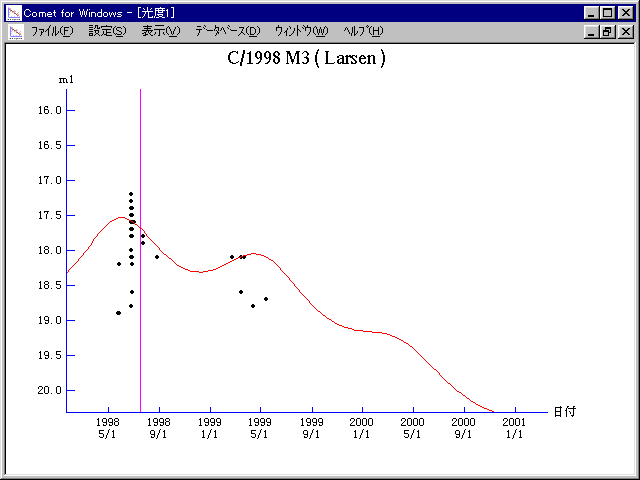# \$B%i!<%;%sWB@1(B

C/1998 M3 ( Larsen )###\$B%W%m%U%#!<%k(B

 \$BH/8+F|(B 1998\$BG/(B6\$B7n(B24\$BF|(B \$BH/8+8wEY(B 17.6\$BEy(B \$BH/8+ J. Larsen

###\$B###\$B50F;MWAG(B

```   The following improved orbital elements by Kenji Muraoka, are
from 104 observations 1998 May 26 to 1999 June 14, perturbations
by 9 Planets, Moon and 5 minor planets were taken into account.
The mean residual is +/- 0.73 arc seconds.

Epoch  =  1998 July  6.0  TT       JDT = 2451000.5
T  =  1998 July 17.09589       +/- 0.01398 (m.e.) TT
Peri. =   20.83628                +/- 0.00133
Node  =  255.52549                +/- 0.00067   (2000.0)
Incl. =  113.42024                +/- 0.00060
q  =    5.7681916              +/- 0.0000354 AU
e  =    1.0022061              +/- 0.0000290
1/a  =   -0.0003825              +/- 0.0000050 1/AU
orig. 1/a  =   +0.000147
fut.  1/a  =   +0.000208
```

###\$B@1?^(B###\$B8wEYJQ2=(B

```        m1 = 6.5 + 5 log\$B&\$(B + 10.0 log r
```##### \$B50F;MWAG\$OB<2,7r<#;a\$N7W;;\$K\$h\$k\$b\$N\$G\$9!#(B \$B@1?^\$O(B StellaNavigator Ver.2.0 for Windows (\$B%"%9%H%m%"!<%D(B \$BJTCx(B / \$B%"%9%-!<=PHG6I4)(B) \$B\$G:n@.\$7\$?\$b\$N\$G\$9!#(B \$B8wEY%0%i%U\$O(BComet for Windows\$B\$G:n@.\$7\$?\$b\$N\$G\$9!#(B